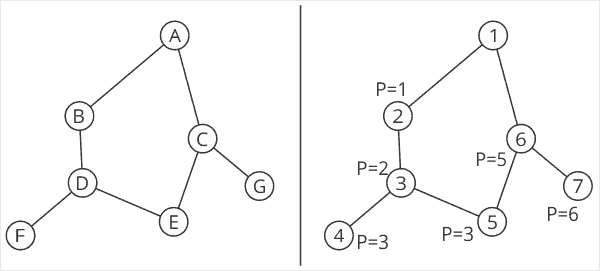# DFS and Low Points

On a recent history of networking episode, Alia talked a little about Maximally Redundant Trees (MRTs), and the concept of Depth First Search (DFS) numbering, along with the idea of a low point. While low points are quickly explained in my new book in the context of MRTs, I thought it worthwhile to revisit the concept in a blog post. Take a look at the following network:On the left side is a small network with the nodes (think of these as routers) being labeled from A through G. On the right side is the same network, only each node has been numbered by traversing the graph, starting at A. This process, in a network, would either require some device which knows about every node and edge (link) in the network, or it would require a distributed algorithm that “walks” the network from one node to another, numbering each node as it is touched, and skipping any node that has already been visited (again, for more details on this, please see the book).

Once this numbering has been done, the numbers now produce this interesting property: if you remove the parent of any node, and the node can still reach a number lower than its own number, the network is two-connected. Take E, numbered as 5, as an example. E’s parent is D, labeled as 3 on the numbered side of the illustration. If you remove D from the network, what is the lowest numbered node E can reach? Start by jumping to the lowest numbered neighbor. In this case, E only has one neighbor remaining, C, which is numbered 6. From here, what is the lowest numbered neighbor of C? It is A, with a number of 1.

D, then, can reach a node which is numbered 1 through some other neighbor than its parent. This means D has some other path to the parent than through its parent, which means D is part of a topology with at least two connections to some other node in the network—it is two connected.

Using this sort of calculation, you can find alternate paths in a network. The problem with using DFS numbering for this is what was stated above—the calculation requires either a “walk through the network” protocol, or it requires some device with a complete view of the network (an LSDB, in link state terms). Neither of these are really conducive to real time calculation during a topology change. MRT solves this by using low points from DFS numbering with Dijkstra’s SPF algorithm to allow the calculation of disjoint paths in near real time in a distributed control plane.